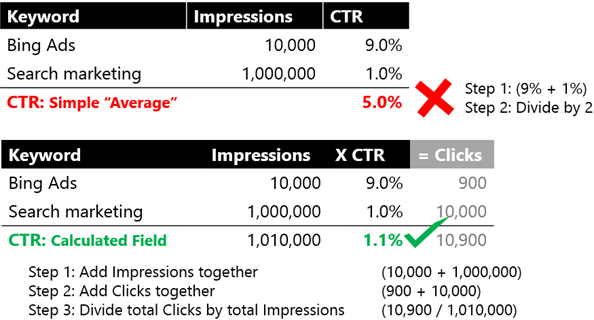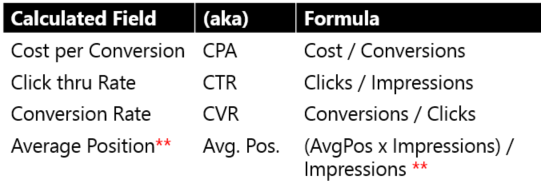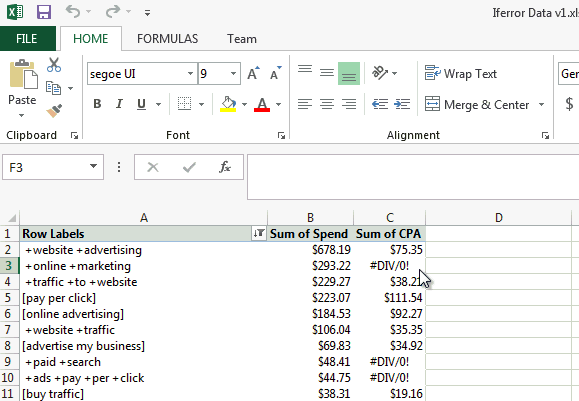2 Essential Pivot Table Skills for Marketers: Summarizing Data and Calculated FieldsWe’ve previously covered a few basics for pivot tables, a powerful Excel tool which helps digital marketers organize and understand data. Today we’re going a half-step beyond the basics of setting up a pivot table, by exposing “must-know skills” for digital marketers.

You must know how to use these two essentials in order to get a different perspective on your marketing data through the pivot table function:

• Summarizing Data
• Calculated Fields

If you’ve been using pivot tables for years, you’ll still probably find a few “ah-ha” moments here, like how to get rid of the pesky “GETPIVOTDATA()” formula.

1. Summarize Data: The First Step, and Often First MIstake

You poured data into a pivot table. Now what? The very first thing you must do is make sure the data is summarized correctly.

There are several ways to summarize your data, but “Sum” and “Count” are most common in digital marketing. Here’s how they’re commonly used:

• “Sum” is used for data like visits, revenue, conversions, clicks, spend, and impressions.
• “Count” can be used for counting the number of keywords, pages, geographic locations, or campaigns.

For search marketers, the most common mistake is summarizing data using the “Average” function in pivot tables. Instead of “Average”, we actually need to use a feature in Excel called Calculated Fields.

TIP: Take a closer look at “Average” in pivot tables.

So why doesn’t “Average” work? Let’s walk through a simple scenario comparing how using “Average” reports vastly different data.

We have two keywords in our ad group; a branded term and a generic term. One keyword has lots of impressions and a lower CTR, the other has fewer impressions but a high CTR. See the example below:A 5 percent CTR vs. a 1.1 percent CTR is vastly different. Turns out an “Average” calculation from pivot tables provides 5 percent CTR, which would track back to 50,500 clicks vs. the 10,900 clicks actually received. Clearly incorrect.

2. Calculated Fields: Use Smarter Math for Accuracy

To get this more accurate calculation, you’ll need to use Calculated Fields. A calculated field is quite simple: you select a column of data (also known as a “field”) and tell Excel a specific calculation you’d like to do.

Here are some examples of common calculations you’ll make in digital marketing:**Note: Average Position is a weighted average. You’ll first need to add a new column in your data sheet, named “AvgPos x Impressions” above, the column will multiples Avg. Position x Impressions. See more here.

Of all the useful techniques you learn for pivot tables, the Calculated Fields function is the ultimate treasure. As a general rule, anything that involves an average or percent (CTR, CPA, CRV, CPI or Avg. Position) will require a formula created through the Calculated Fields dialog box instead of with the pivot tables Summarize Data dialog box.

Whatever you’re focusing on in your pivot table, Calculated Fields allows you to do the math behind the scenes. Without Calculated Fields, marketers have to copy and paste their pivot tables and then copy their formulas an endless number of times. This is how you get innocent mistakes in your data.

See more dynamic Calculated Field tutorials here.

Tip: Use IFERROR() in Calculated Fields as a Cue

A problem frequently experienced using Calculated Fields is the #DIV/0! error (which means you can’t divide by zero). This can happen when calculating CPA (ad spend/conversions), for instance, if you had no conversions (0).

If you ignore the error, you’re missing out on valuable information. For example, keywords can spend thousands and convert zero times.

This is information you’ll want when you sort from worst CPA to best. But it won’t show up if you’ve left the #DIV/0! error untouched.

The solution to this problem is adding the IFERROR() formula to the calculated field. Add the IFERROR() formula and make sure to return “spend” or “cost”, as shown below. Open the Calculated Fields dialog again, go to the CPA field, and change the formula to:

=IFERROR(Spend/Conversions,Spend)Turns out IFERROR() isn’t the only formula you can use in calculated fields.

Tip: Get Rid of GETPIVOTDATA()

Bill Jelen (aka Mr. Excel) said it best, “You’ve just built a pivot table in Excel. You click outside of the pivot table. You build an Excel formula. You copy this formula down to all of the rows in the pivot table. The calculation reports the wrong answer for all but the first row of the pivot table. You’ve just been stung by the Generate GetPivotData ‘feature’.”

We’ve all been there. You’re expecting to see =(C3/D3). That is nothing like the mess that shows up. You can fix this mess by simply turning of GETPIVOTDATA.

Here is how you can turn off GETPIVOTDATA.

How is this feature useful? Think of GETPIVOTDATA like the GPS coordinates for data in your table.

In pivot tables, data moves around frequently and quickly. GETPIVOTDATA keeps tabs on your data, so you can build charts and other formulas as your data moves around (as long as your data is visible).

All that GPS data can also turn simple calculations into a ridiculous cascading mess. Turning off GETPIVOTDATA lets you do simple, quick calculations without dragging the entire hemisphere into the matter. It frees you up to move more nimbly when you need to.

More Tips, Next Time

We’ll break down sorting and filtering in the next part of this series, outlining one or two more tips you may not have known. Was this helpful? Anything to add? Any questions? Please let me know and use the comments below!